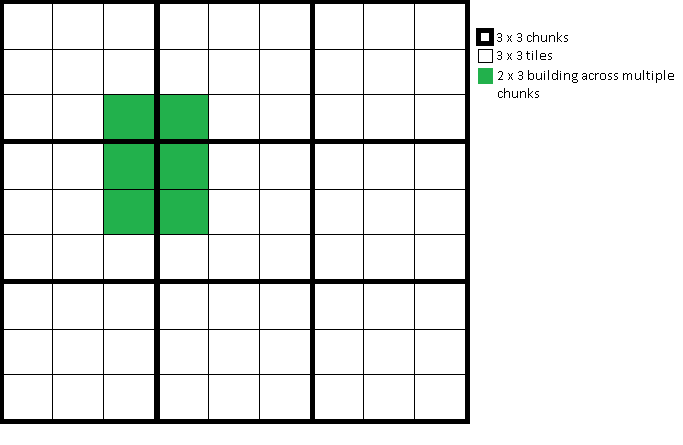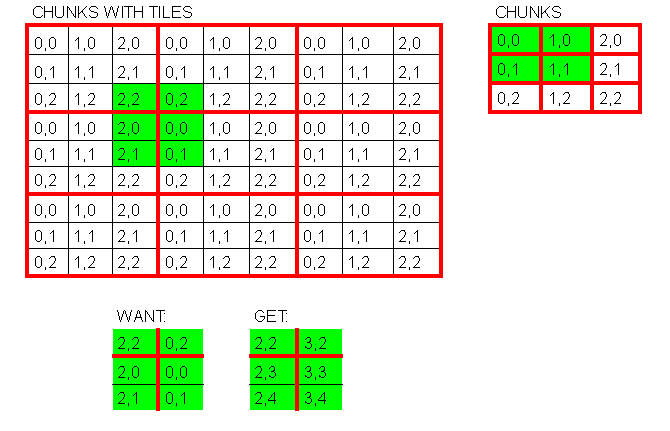# C# Fastest way to iterate over certain tiles overlapping multiple chunks?

What's the fastest way of iterating over certain tiles overlapping multiple chunks in C#.

Ideally without having to iterate over everything, just a specific set of coordinates? (green cubes example below)

I had some partial solutions, but i'd like to see what you guys come up with.

For example:

I would like to set every tile.IsBuiltOn = true for every tile beneath the building.

I could also use this to check if tile.IsBuiltOn = true to refuse placement of another building.

Chunks and each array of tiles of chunks start at [0,0].class World
{
public Chunk[][] Chunks { get; set; }
}

class Chunk
{
public Tile[][] Tiles { get; set; }
public Dictionary<CoordinateKey, Building> Buildings { get; set; }
}

class Building
{
public BuildingID BuildingID { get; set; }
public CoordinateKey ChunkCoordinate { get; set; }
public CoordinateKey TileCoordinate { get; set; }
public int WidthInTiles { get; set; }
public int HeightInTiles { get; set; }
}

class Tile
{
public bool IsBuiltOn { get; set; } = false;
}


CoordinateKey is just a struct with int X, Y.

Here is my solution.

First I created a Google sheets to help me map out the chunks and tiles with their coordinates, what I want and what I get from my current iteration code:I want to iterate over the green tiles, across multiple chunks and receive the correct tile coordinates and chunk coordinates, with only the building Width, Height, start tile X,Y and chunk start X,Y.

This allowed me to finally come up with a working solution (below), which is fast.

Note: To get this solution working quickly I have a lot of code in class constructors, this is not advisable and I will refactor later when I move this solution into my main project.

I used https://csharppad.com/ to quickly test the following code, which can be pasted in the "interactive shell" panel, then click "Go":

class World
{
public Chunk[][] Chunks;
public int ChunkSizeTilesXY = 3;

public World()
{
Chunks = new Chunk[];

for (int x = 0; x < 3; x++)
{
Chunks[x] = new Chunk;

for (int y = 0; y < 3; y++)
{
Chunks[x][y] = new Chunk()
{
chunkX = x,
chunkY = y
};
}
}

Test();
}

private void Test(){

int buildingWidthInTiles = 2;
int buildingHeightInTiles = 3;

int startTileX = 2;
int startTileY = 2;
int endTileX = startTileX + buildingWidthInTiles;
int endTileY = startTileY + buildingHeightInTiles;

int startChunkX = 0;
int startChunkY = 0;

int chunkX = startChunkX;
int chunkY = startChunkY;
int ctx = startTileX;
int cty = startTileY;

for (int tx = startTileX; tx < endTileX; tx++)
{
// Out of bounds of current chunk, increment chunk and ctx (chunk tile x)
if (ctx > ChunkSizeTilesXY-1)
{
chunkX++;
ctx = 0;
}

for (int ty = startTileY; ty < endTileY; ty++)
{

// Out of bounds of current chunk, increment chunk and cty (chunk tile y)
if (cty > ChunkSizeTilesXY-1)
{
chunkY++;
cty = 0;
}

Chunks[chunkX][chunkY].Tiles[ctx][cty].IsBuiltOn = true;

Console.WriteLine("tx:" + tx + ", ty: " + ty + ", ctx:" + ctx + ", cty: " + cty + ", chunkX: " + chunkX + ", chunkY:" + chunkY);

cty++;

}

ctx++;

// Reset chunkY
chunkY = startChunkY;

}

// Reset chunkX
chunkX = startChunkX;

}

}

class Chunk
{
public Tile[][] Tiles;
public int chunkX;
public int chunkY;

public Chunk()
{
Tiles = new Tile[];

for (int x = 0; x < 3; x++)
{
Tiles[x] = new Tile;

for (int y = 0; y < 3; y++)
{
Tiles[x][y] = new Tile()
{
chunkX = chunkX,
chunkY = chunkY
};
}
}
}

}

class Tile
{
public bool IsBuiltOn = false;
public int chunkX;
public int chunkY;

public Tile()
{

}

}

var world = new World();


I am still interested in hearing from others on alternate or faster solutions.

• This is basically how I would do it. There are a few code conventions that are a bit off but this isn’t codereview se and the concept is right. Jun 30, 2018 at 12:32
• @EdMarty Thanks for your feedback. Please by all means feel free to suggest small changes/improvements, I may submit this to codereview se at a later date for a thorough review. One thing I will be doing though is moving all the code from the constructors into separate methods. Jun 30, 2018 at 12:56

Your solution is close; what you should do is divide each coordinate to find which chunk each coordinate is in.

enum POSITION_TYPE
{
TOP_LEFT,
TOP_RIGHT,
BOTTOM_LEFT,
BOTTOM_RIGHT
};

TILE_WIDTH = 3;
for each coordinate
findChunk(Math.Floor(coordinate.X / TILE_WIDTH),
Math.Floor(coordinate.y / TILE_WIDTH));
markBuilt(coordinate.PositionType(),
coordinate.X % TILE_WIDTH, // finds offset into local coordinates of chunk
coordinate.Y % TILE_WIDTH, // finds offset into local coordinates of chunk


Each position type requires a different algorithm of filling squares, but they're all simple alterations (and it could possibly dealt away with with clever use of negatives or positives possibly.)

What this does is find which chunk you're in by realizing that:

• you're always a positive number
• Your tile-width is constant, so dividing by 3 gives you your chunk given a coordinate
• Your tile-local-grid is figured out by the modulus (the remainder of a divide) of Tile Width, for the same reasons as above

NOTE: Given that you'll only be marking up the grid when a building is created or destroyed, the divides/modulus aren't so bad. You may consider some hackery to avoid them; such as "x * .33" if you're using floats or moving to 2x2 or 4x4 super-tiles so that you can use bit-shifting to perform your divide for you (in the case of longs or ints.)

• Thanks for your solution, I like your use of the modulus operator and reducing 2 loops into 1. Jun 30, 2018 at 13:00